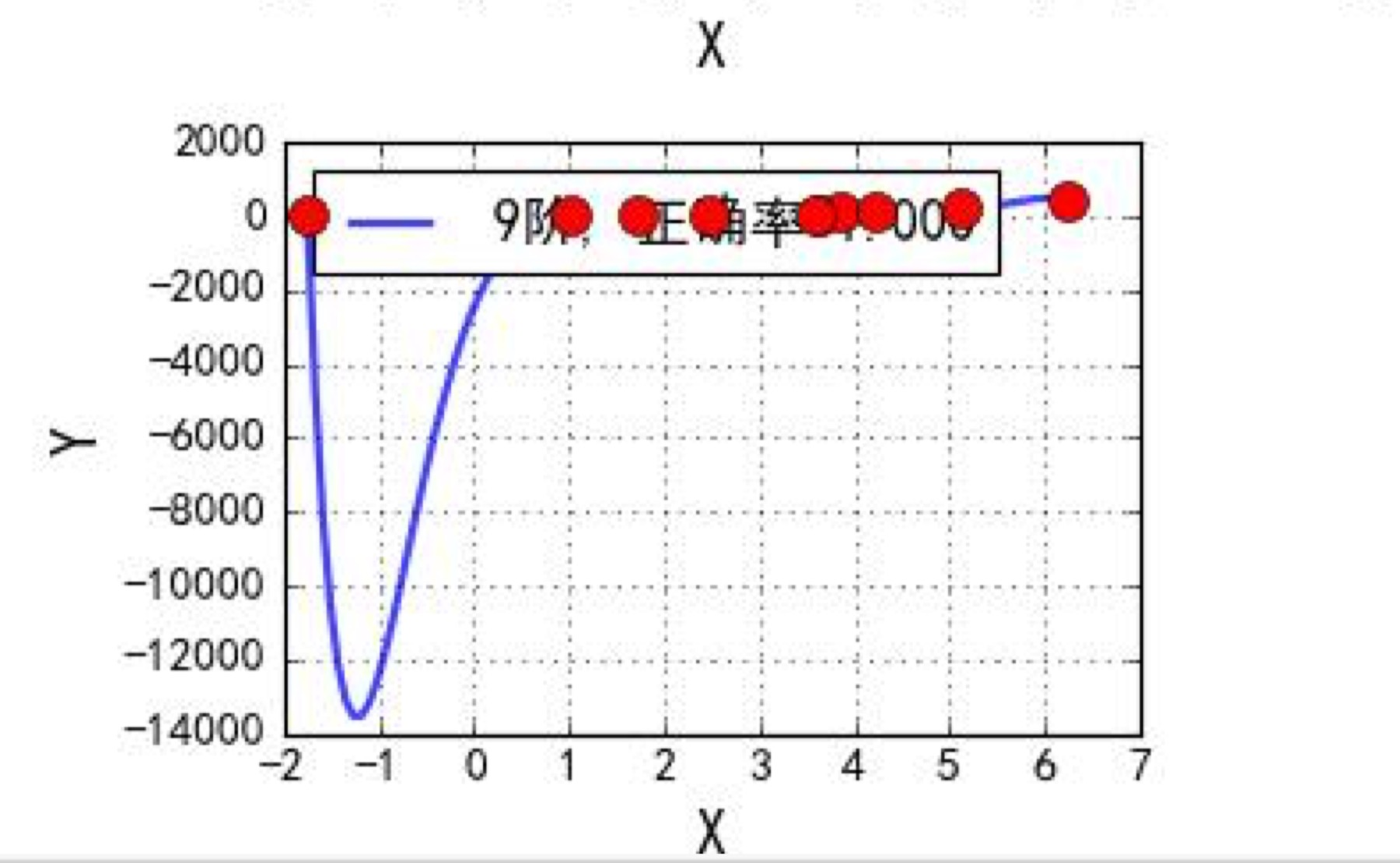## 回归算法

​在序章中我们提到了，机器学习的本质就是一个分类器，对给出的数据进行有价值的分类。 ​具体的机器学习算法的分类分为，监督学习和无监督学习两种。而在监督渡学习中，我们以分类的类别是否是离散的，分为两种分类方式，分别是分类回归。即，分类后是有一定的，像水果的分类，苹果，梨，橘子等等这样确定的分类的是分类，而分类后的预测结果是一个连续的数值则是回归。在这篇文章中，我们说的回归算法便是监督算法中的回归算法。

## 最小二乘参数&损失函数

​还记得我们最开始说的，机器学习的实质就是分类么，即得到输入$x$后，通过一个训练好的关系$f(x)$，输出一个$\hat y$ ，如果你的模型好的话，$\hat y$ 和真实值$y$会非常接近，有着非常好的预测结果。在这一个章节中，将会从数学层面来讲一讲这个是如何做到的。 首先我们用大白话，整体的了解下这是一个什么样子过程。首先，我们需要先确定个目标，打比方说我要做一锅好吃的菜，我刚开始拿到一个方子，买来了菜切好了菜，按照方子做了，即选了算法，拿到训练数据后，机器学习第一次迭代。之后，尝了一尝，觉得这个菜不和我胃口，即和已有数据标签对比，然后调整盐量，菜的切法之后，即发现结果不理想，以某种方式调整参数，再做一次，做完之后再尝，再调整，即再次迭代，最终研究出了自己喜欢的菜适合自己的配方，即符合要求的准确率停止训练

​看这个过程是不是简单的说就是，不断的调整方法，直到完成目标任务，就和小婴儿学习一样。那么过程现在知道了，那如何让我们的小婴儿计算机来学习呢。首先，我们想，最简单的方程是什么呢，很小我们就知道了$y = kx + b$ 这个直线公式，我们现在就用它来作为输入$x$和输出$y$之间的关系，所以引入下面的公式2.1。我是这么理解的，每一个输入 $x$ 都对输出 $y$ 有不同的作用效果，所以我们给输入 $x$ 前面写一个系数来代表它的作用强度，这个系数就是$k$ ，也就是下面公式中的 $\theta$ ， 那么后面的$\epsilon$ 是什么呢。我的理解是，你看，我们现在是做一个$y$ 的预测，从实际出发，我们很难找到完美的$\hat y$ 可以完全等于训练集中$x$ 所对应的 $y$ ，这时候误差是必定存在的，所以引入误差$\epsilon$，为我们通过模型预测的值$\hat y$ 和已知值$y$的差，如公式2.2所示。除此之外，我们可以把$\epsilon$ 看作现实中众多随机现象引起的误差，而这个误差一般符合高斯分布（参考中心极限定理），这个在之后的推导中也会用到。实际问题中，很多随机现象可以看做众多因素的独立影响的综合反应，往往服从正态分布。

$y^{(i)} = \theta^T*x^{(i)}\ \ \ 公式2.1 ​$ $y^{(i)}- \theta^T*x^{(i)}= \epsilon^{(i)}\ \ \ 公式2.2$

​误差、误差，顾名思义，我们要让误差最小，由于$\epsilon$符合高斯分布（最大似然估计），所以$\epsilon$ 的概率应该符合公式2.3。然后用公式2.2进行等式代换得到公式2.4。那么这个公式2.4怎么理解呢，这里输入$x$是固定的，我们只能调整$\theta$来使输出$\hat y$来接近样本给出的$y$。而公式2.4的意思就是，在输入$x$的情况下，输出正确$y$的概率。

$p(\epsilon^{(i)}) = \frac{1}{\sigma \sqrt{2\pi}}e^{-\frac{(\epsilon)^2}{2 \sigma^2}}\ \ \ 公式2.3$ $p(y^{(i)}|x^{(i)};\theta) =\frac{1}{\sigma \sqrt{2\pi}}e^{-\frac{(y^{(i)}- \theta^T*x^{(i)})^2}{2 \sigma^2}}\ \ \ 公式2.4$

​接下来我们要引入最大似然估计，小伙伴你们没有听错，就是考研数学中概率论的那个最大似然估计（居然真的用上了）。由于每个输入都是相互独立的，所以整体预测对的概率密度，可以通过每个样本预测对的概率密度相乘得到。于是我们得到了下面的公式2.5。是不是很熟悉，我们现在做的是改变 $\theta$ 使得概率最大，就和考研的时候学的一样，我们对公式2.5先求对数，然后求导就可以算出来我们要的 $\theta$ 的。

$L(\theta) = \prod_{i = 1}^mp(y^{(i)}|x^{(i)};\theta)\ \ \ 公式2.5$

​在这里有一点小小的变化，为了方便计算，我们对公式2.5求对数以后，我们得到了公式2.6。观察公式2.6我们很快的发现。$m \log \frac{1}{\sigma \sqrt{2\pi}}$ 和 $m \log \frac{1}{\sigma \sqrt{2\pi}}$都是常数，和$\theta$ 相关的只有后面那部分。所以我们求导光求导后面的那块就好了。然后就可以得到一个 $\theta$ 和输入和输出的关系公式2.7，机器就是这么求出最优解的，但是矩阵的求逆是很复杂的，这里会耗费大量的计算量。

$\ell(\theta) = m \log \frac{1}{\sigma \sqrt{2\pi}} - \frac{1}{\sigma^2}*\frac{1}{2}\sum^m_{i =1}(y^{(i)}-\theta ^{T}x^{(i)})^2 \ \ \ 公式2.6$

$$\theta = (X^TX)^{-1}X^TY \ \ \ 公式2.7$$ ​ps. 此处公式的求解为最小二乘法，可以自行百度下

​最后，我们在公式2.6中看到一个很有意思的公式，这个只要是了解过损失函数的人都是眼熟的。这个公式就是 $-\frac{1}{2}\sum^m_{i =1}(y^{(i)}-\theta ^{T}x^{(i)})^2$ ，回想下损失函数的作用是什么，是一个求 $\theta$ 的一个关键函数，它代表着预测的是不是准确，然后根据这个函数来调整我们通过输入 $x$ 生成 $y$ 的函数 $h(x)$。所以我们可以写出如下公式2.8，而这个正是常用损失函数中的平方和损失函数。 $$loss = J(\theta) = \frac{1}{2}\sum^m_{i =1}(h_{\theta}(x^{(i)})-y^{(i)})^2 \ \ \ 公式2.8$$

​实际中我们有很多损失函数，如 0-1 损失函数感知损失函数平方和损失函数绝对值损失函数对数损失函数

## 3、多项式扩展

​现在我们对整体的流程也有了了解，这里讨论下，在之前讨论中输入输出关系 $f(x)$ 的一些遗留问题。之前我们说我们的目的就是找到这么一个 $f(x)$，但是这个关系不会凭空出现，所以我们预测了一个关系 $h(x)$，在预测的时候我们给每个样本属性 $x$ 前都乘上了一个系数 $\theta$，但是这么做有一个前提就是，这些属性之间是相互没有关联的，而这恰恰与实际完全不符。解决这个问题的正是，对函数 $h(x)$ 进行多项式扩展，多项式扩展后出现了属性之间相乘的形式，简单的来说就是将原本分离的 $(x_1+x_2)$，通过给他们平方得到 $(x_1^2 + 2x_1x_2+ x_2^2)$，显然就表示了属性间的相关性，预测的准确率也就大大提升了。

## 4、什么是过拟合&过拟合问题的解决

​在我的理解中，即便是机器学习也是按照人指的方向进行数学问题的求解操作。在这个机器学习中，我们一定会找到一个通过样本来看预测效果非常非常好的结果，但是这个效果真的好么。如下图，我们可以看到，每一个样本都是符合的，但是很显然这个曲线是不对的。​这是什么原因导致的呢，在通过输出 $\theta​$ 值，我们发现，这是由于某些 $\theta​$ 值过大导致的。所以我们由此可以想到解决方案就是，用一个添加项来迫使$theta​$不至于过大。在这里我们引入正则项（norm），即L1-normL2-norm。通过公式我们可以看出，我们要让损失函数很小，有了正则项的抑制，势必 $\theta​$ 不会变得太大，毕竟损失函数的结果和 $\theta$ 的值挂钩了。

L2-norm：$J(\theta) = \frac{1}{2}\sum^m_{i=1}(h_{\theta}(x^{i}) - y^{(i)})^2 + \lambda\sum^n_{j=1} \theta_j^2 \ \ \ \lambda > 0$

L1-norm： $J(\theta) = \frac{1}{2}\sum^m_{i=1}(h_{\theta}(x^{i}) - y^{(i)})^2 + \lambda\sum^n_{j=1} |\theta_j| \ \ \ \lambda > 0$

​当我们使用L2-norm的线性回归模型就是Ridge回归（岭回归模型），而我们使用了L1-norm 的模型则是LASSO回归。接下来我们分析下这两个的性能问题。

​L2-norm 中，由于对于各个维度的参数缩放是在一个圆内缩放的，几乎不可能导致有维度参数变为 0 的情况，那么也就不会产生稀疏解；而 L1-norm 是在一个方形内的，则很容易产生稀疏解。实际应用中，数据的维度中是存在噪音和冗余的，稀疏的解可以找到有用的维度并且减少冗余，提高回归预测的准确性和鲁棒性（减少了过度拟合），而 L1-norm 则可以达到最终解的稀疏性的要求。 ​所以，Ridge 模型有较高的准确性和鲁棒性，而 LASSO 模型更快，更能晒出稀疏解。那么如果我们两个属性都要兼备怎么办呢。接下来就引入了弹性网络ElasitcNet算法。其实就是很暴力的同时引入了 L1-norm 和 L2-norm，然后用$p$来代表哪个多点，具体公式如下。

$J(\theta) = \frac{1}{2}\sum^m_{i=1}(h_{\theta}(x^{i} - y^{(i)}))^2 + \lambda(p\sum^n_{j=1} \theta_j^2 +(1-p)\sum^n_{j=1} |\theta_j| )\ \ \ \lambda > 0 \&\& p \in [0,1]$
• 本文作者： Author：DeamoV
• Github：https://github.com/Duan-JM
• Email：vincent.duan95@outlook.com
• 本文链接： Artical： 线性回归算法
• 版权声明： 本博客所有文章除特别声明外，均采用 CC BY-NC-SA 3.0 许可协议。转载请注明出处！
• 版权声明： 原创文章如转载，请注明出处